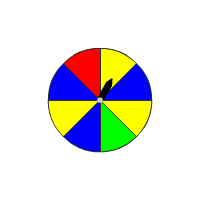# Using Probabilities to Make Fair Decisions

A probability experiment may be considered "fair" if all outcomes are equally likely, or (in some cases) if the expected value of some random variable is $0$ .

Example 1:

There are $6$ players in volleyball game. The team has to choose one of them randomly to be captain for a game.

Tasha's plan : Assign each player a number. Then roll a number cube. The captain is the player whose number comes up.

Martin's plan : Assign each player a number. Then flip $3$ coins. Select a player according to the following chart.

Check whether both the plans can be considered fair in selecting a captain.

First check Tasha's plan for fairness.

The sample space of the number cube is $\left\{1,2,3,4,5,6\right\}$ and each is equally likely possible outcome.

Each player has equal chance of selection as captain with probability of $\frac{1}{6}$ .

Next check Martin's plan for fairness.

The sample space of flipping $3$ coins is and there are $8$ outcomes, which are equally likely.

The players $1$ and $2$ have probability of $\frac{2}{8}$ to be selected as captain, whereas the other players have probability of $\frac{1}{8}$ . Here, each does not have equal chance of selection as captain.

So, Martin's plan cannot be considered to be "fair" in the selection of captain.

Example 2:

At a school fair, you are given a number of tokens. In one stall at the fair, there is a spinner with $8$ sectors. If the spinner lands on a red sector, you win $3$ tokens. If you land on a green sector, you win $5$ tokens. If you land on any other sector, you lose $2$ tokens.

Is this game fair?The spinner has $8$ sectors and each is equally likely possibility.

Sample space is {red sector, green sector, $6$ other sectors}

Write the probability distribution for a single spin of spinner and the amount of tokens you win.

$\begin{array}{|cccc|}\hline x=\text{color}& \text{Red}& \text{Green}& \begin{array}{l}\text{Others}\\ \left(\text{Blue}\text{\hspace{0.17em}}\text{\hspace{0.17em}}\text{and}\text{\hspace{0.17em}}\text{\hspace{0.17em}}\text{Yellow}\right)\end{array}\\ P\left(x\right)& \frac{1}{8}& \frac{1}{8}& \frac{6}{8}\\ \text{Tokens}& 3& 5& -2\\ \hline\end{array}$

Use the weighted average formula.

$\begin{array}{l}E\left(x\right)=3\cdot \frac{1}{8}+5\cdot \frac{1}{8}+\left(-2\right)\cdot \frac{6}{8}\\ =\frac{3}{8}+\frac{5}{8}-\frac{12}{8}\\ =-\frac{4}{8}\text{\hspace{0.17em}}\text{\hspace{0.17em}}\text{or}\text{\hspace{0.17em}}\text{\hspace{0.17em}}-0.5\end{array}$

The expected value is not zero, and the game is not fair. So you will lose about $0.5$ tokens for a single spin.